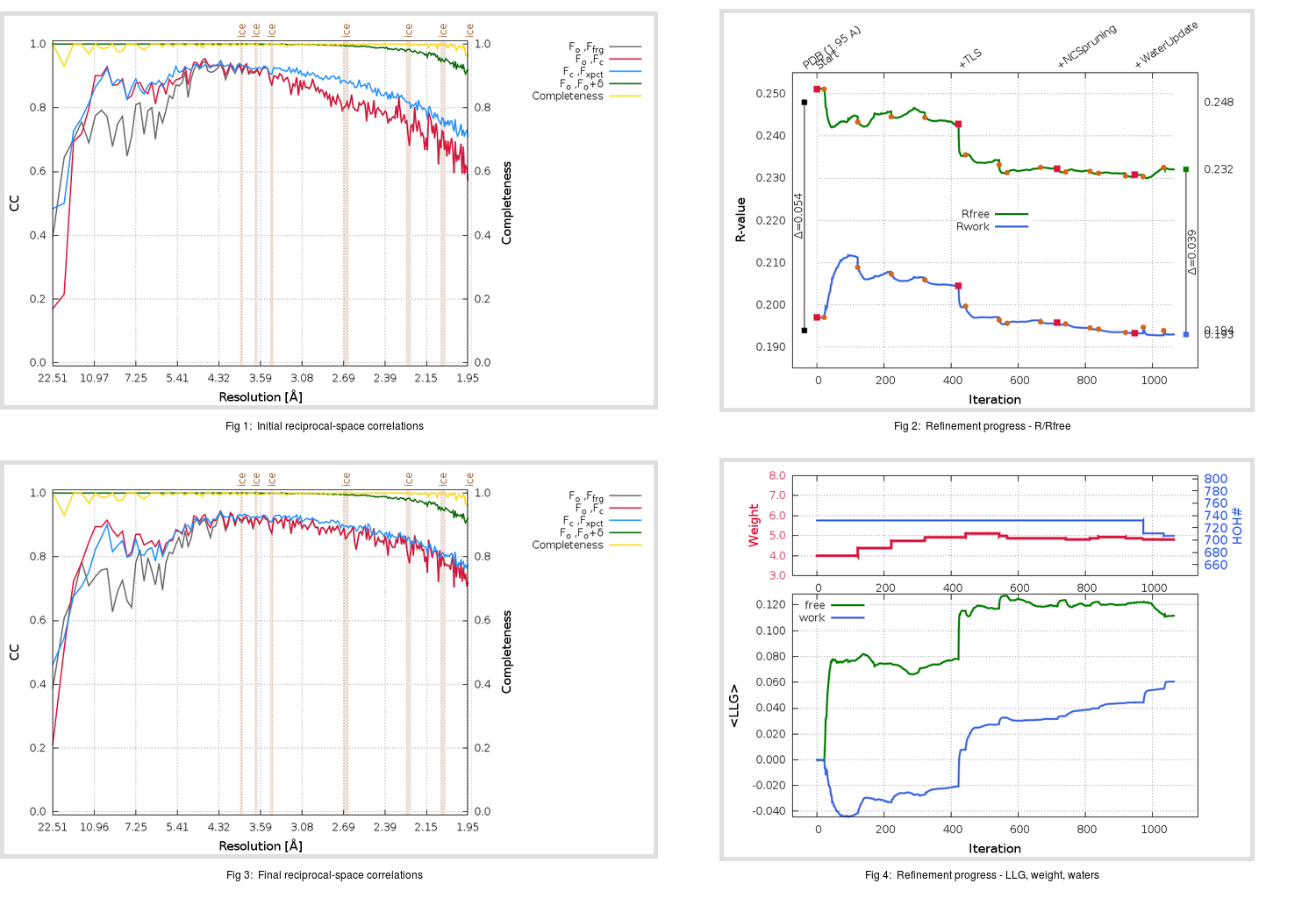Content:

```    Diffraction limits & principal axes of ellipsoid fitted to diffraction cut-off surface:
1.907         1.0000   0.0000   0.0000       0.956 a* - 0.292 c*
1.920         0.0000   1.0000   0.0000       b*
1.918         0.0000   0.0000   1.0000       c*
```

## Deposited

` `
 Date deposited Date data collection Resolution R, Rfree 20070920 20051112 1.95 0.1940 0.2480

Molprobity (CCP4 7.0 version) summary:

```Ramachandran outliers =   0.17 %
favored =  96.51 %
Rotamer outliers      =   3.85 %
C-beta deviations     =     0
Clashscore            =  14.26
RMS(bonds)            =   0.0092
RMS(angles)           =   1.67
MolProbity score      =   2.33
Resolution            =   1.95
R-work                =   0.1940
R-free                =   0.2480
```

```Number of waters      =   732

<B> (all atoms) =   31.28 ( sd =    9.73 ) for       5440 non-hydrogen atoms
<B>   (protein) =   29.68 ( sd =    8.84 ) for       4686 non-hydrogen atoms
<B>     (water) =   40.88 ( sd =    8.80 ) for        732 non-hydrogen atoms
<B>    (others) =   53.08 ( sd =    8.98 ) for         22 non-hydrogen atoms

B min/max       (all non-hydrogen atoms) =   14.31 /   67.82
B min/max   (protein non-hydrogen atoms) =   14.31 /   60.51
B min/max     (water non-hydrogen atoms) =   17.67 /   67.82
B min/max     (other non-hydrogen atoms) =   36.69 /   64.85
```

## BUSTER (re-)refinement

` `

Molprobity (CCP4 7.0 version) summary:

```Ramachandran outliers =   0.00 %
favored =  98.67 %
Rotamer outliers      =   3.65 %
C-beta deviations     =     2
Clashscore            =   5.04
RMS(bonds)            =   0.0116
RMS(angles)           =   1.55
MolProbity score      =   1.69
Resolution            =   1.95
R-work                =   0.1930
R-free                =   0.2321
```

```Number of waters      =   707

<B> (all atoms) =   32.74 ( sd =   12.74 ) for       5415 non-hydrogen atoms
<B>   (protein) =   30.43 ( sd =   10.61 ) for       4686 non-hydrogen atoms
<B>     (water) =   47.01 ( sd =   13.74 ) for        707 non-hydrogen atoms
<B>    (others) =   65.32 ( sd =   33.83 ) for         22 non-hydrogen atoms

B min/max       (all non-hydrogen atoms) =   14.54 /  146.81
B min/max   (protein non-hydrogen atoms) =   14.54 /   82.89
B min/max     (water non-hydrogen atoms) =   16.29 /  110.13
B min/max     (other non-hydrogen atoms) =   39.55 /  146.81
```

Refinement progression:Results:

` `
 File Remark 2Z9J_aB_refine.01_04_refine.pdb.gz exact refinement commands are in header 2Z9J_aB_refine.01_04_refine.mtz.gz including original deposited data and several re-refinement map coefficients 2Z9J_aB_refine.01_04_BUSTER_model.cif.gz including any non-standard compound restraints 2Z9J_aB_refine.01_04_BUSTER_refln.cif.gz